## 参数设置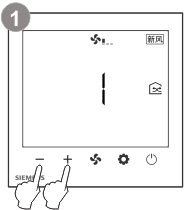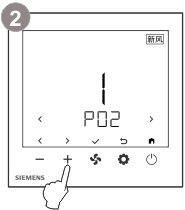或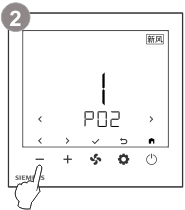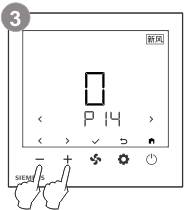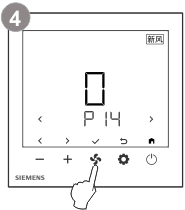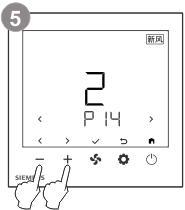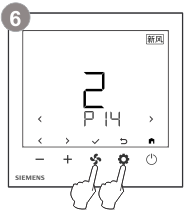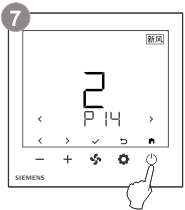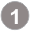同时按下 + 和 - 键 3 秒以上。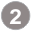初级参数：松开按键且在 2 秒内按下 + 键，直到屏幕显示 Pxx。高级参数：松开按键且在 2 秒内按下 - 键，直到屏幕显示 Pxx。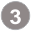按下 + 或 - 键（使用 < 或 > 键）选择参数，所选参数的当前值会显示。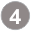按下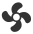键（使用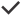键）以编辑所需参数的值。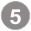在编辑过程中，按下 + 或 - 键增加/减少或选择当前参数的值。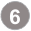按下键（使用键）以确认更改的值，或按下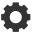键（使用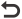键）取消更改。重复步骤 3 到 5，以更改更多参数。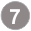按下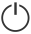键（使用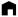键）退出参数设置模式。

## 恢复出厂设置

• 选择参数 P71，并把参数值设置为 1。
• 控制参数的出厂设置将会重新加载。
• 重载过程中，屏幕显示“- - -”。

## 控制参数

P02 *

1

1 = 开启（ON） – 关闭（OFF）

2 = 开启（ON） – 节能（ECO） – 关闭（OFF）

P14

0

0 = 解锁

1 = 仅风速可调

2 = 全锁

P24 *

0

0 = 禁用

1 = 启用

P25 *

0

0 = 禁用

1 = 启用

P26

1

1 = 1:5

2 = 2:4

3 = 3:3

4 = 4:2

5 = 5:1

P27

0

0 = 前一运行模式

1 = OFF （关闭）

2 = ON（标准模式）

P28

1

0 = 禁用

1 = 启用

* 这些参数至关重要，必须根据调试期间选择的设备进行设置。

P54 *

3

1 = 1 速风机

2 = 2 速风机

3 = 3 速风机

4 = DC 0...10 V（ECM 风机）

P55

ECM 风机最大输出

80 %

ECM 风机最小值…100 %

P56

ECM 风机最小输出

30 %

1 %...ECM 风机最大值

P59

2 min

1…6 min

P71

0

0 = 禁用

1 = 开始重载

P98 *

1

0 = 禁用

1 = 启用

* 这些参数至关重要，必须根据调试期间选择的设备进行设置。

d06

d07

0 = 0 % 输出

1...100 = 1 % 至 100 % 输出

P54

d08

Q1 至 Q5 是常开继电器

0 = 所有继电器断开

1 = 仅闭合 Q1

2 = 仅闭合 Q2

3 = 仅闭合 Q3

4 = 仅闭合 Q4

5 = 仅闭合 Q5

6 = 所有继电器闭合

P24、 P25、P54

d09

FAU – 激活旁路风阀

OPE = 打开（正常位置）

CLO = 关闭

P24

d10

FAU – 激活 EXT/INT 循环风阀

OPE = 打开（正常位置）

CLO = 关闭

P25